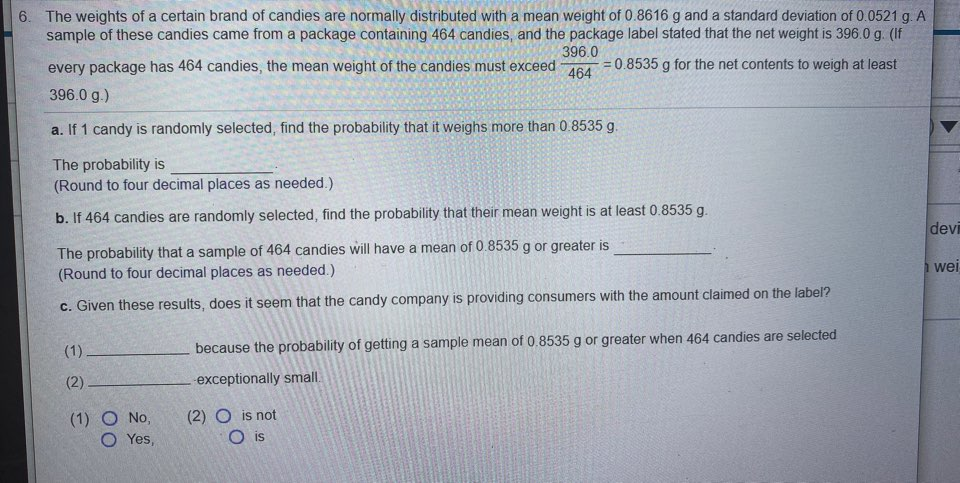# The weights of a certain brand of candies are normally distributed with a mean weight of 0.8616 g and a standard deviation of 0.0521 g. A sample of these candies came from a package containing 464 candies, and the package label stated that the net weight is 396.0 g. (If 396.0 = 0.8535 g for the net contents to weigh at least every package has 464 candies, the mean weight of the candies must exceed %3D 464 396.0 g.) a. If 1 candy is randomly selected, find the probability that it weighs more than 0.8535 g. The probability is (Round to four decimal places as needed.) b. If 464 candies are randomly selected, find the probability that their mean weight is at least 0.8535 g. The probability that a sample of 464 candies will have a mean of 0.8535 g or greater is (Round to four decimal places as needed.) c. Given these results, does it seem that the candy company is providing consumers with the amount claimed on the label? (1) because the probability of getting a sample mean of 0.8535 g or greater when 464 candies are selected (2) -exceptionally small. (2) O is not O is (1) O No, Yes,

Questionhelp_outlineImage TranscriptioncloseThe weights of a certain brand of candies are normally distributed with a mean weight of 0.8616 g and a standard deviation of 0.0521 g. A sample of these candies came from a package containing 464 candies, and the package label stated that the net weight is 396.0 g. (If 396.0 = 0.8535 g for the net contents to weigh at least every package has 464 candies, the mean weight of the candies must exceed %3D 464 396.0 g.) a. If 1 candy is randomly selected, find the probability that it weighs more than 0.8535 g. The probability is (Round to four decimal places as needed.) b. If 464 candies are randomly selected, find the probability that their mean weight is at least 0.8535 g. The probability that a sample of 464 candies will have a mean of 0.8535 g or greater is (Round to four decimal places as needed.) c. Given these results, does it seem that the candy company is providing consumers with the amount claimed on the label? (1) because the probability of getting a sample mean of 0.8535 g or greater when 464 candies are selected (2) -exceptionally small. (2) O is not O is (1) O No, Yes, fullscreen

### Want to see this answer and more?

Experts are waiting 24/7 to provide step-by-step solutions in as fast as 30 minutes!*

*Response times may vary by subject and question complexity. Median response time is 34 minutes for paid subscribers and may be longer for promotional offers.
Tagged in
Math
Statistics

### Data